``````local_options <- options()
library(sf)
library(dplyr)
library(ggplot2)
library(h3jsr)
# for R < 4, since H3 addresses are handled as strings
options(stringsAsFactors = FALSE)``````

## General information

h3jsr connects Uber’s H3 geospatial library to R, via its transpiled JavaScript implementation,`h3-js`. The library has extensive potential applications in network analysis, trip routing, and geospatial data aggregation. The wrapper functions provided are intended to interface well with the existing R-spatial ecosystem, particularly `sf`.

• Documentation for the core H3 library is at H3’s github page.
• The core library only understands WGS84 coordinates, so multiple projection support is limited. All spatial objects are returned in WGS84, and ideally should be supplied as such. It’s always safer to do your own spatial transformations and verify the results. If spatial data in a coordinate system other than WGS84 is supplied, it is transformed using `sf::st_transform()` and a message is issued.
• For each function in `h3jsr`, the default behaviour is to return data in as simple a structure as is practical, but there is always an option to return a more complex-object containing both input and output data, as appropriate for the function in question.
• This package uses `V8` to interface with `h3-js`. As such, a lot of the overhead for each function call is related to sending data to and from V8 via JSON conversion. Feeding large datasets in often gives faster results than one might expect from the toy examples below. Avoid using these functions in conjunction with e.g. `base::lapply` or `purrr::map` on individual geometries!

## Core Functions

Nine core functions exist - three for translating spatial data into and out of the H3 system, and six information utilities, including an address validity checker.

`point_to_cell()` takes in `sf`-style point data and will return the address each point falls into. You can extract addresses for one resolution or many. This function will also accept a matrix or data frame as input, but this will only work if columns 1 and 2 contain WGS84 longitude and latitude values, respectively.

``````# This is the location of the Brisbane Town Hall:
bth <- sf::st_sfc(sf::st_point(c(153.023503, -27.468920)), crs = 4326)

# where is the Brisbane Town Hall at resolution 15?
point_to_cell(bth, res = 15)

By default, a character vector is returned for a single resolution, and a data frame where multiple resolutions are requested. If `simple = FALSE` and the input object inherits from `data.frame`, a data frame object is returned with a new attribute column for each resolution requested.

``````nc <- st_read(system.file("shape/nc.shp", package="sf"), quiet = TRUE)
nc_pts <- st_centroid(nc)
nc_pts <- st_transform(nc_pts, crs = 4326)
nc_pts <- dplyr::select(nc_pts, CNTY_ID, NAME)

# Give me the address for the center of each NC county at every resolution
nc_all_res <- point_to_cell(nc_pts, res = seq(0, 15), simple = FALSE)
#>   CNTY_ID        NAME h3_resolution_0 h3_resolution_1 h3_resolution_2
#> 1    1825        Ashe 802bfffffffffff 812abffffffffff 8244dffffffffff
#> 2    1827   Alleghany 802bfffffffffff 812abffffffffff 8244dffffffffff
#> 3    1828       Surry 802bfffffffffff 812abffffffffff 822a8ffffffffff
#> 4    1831   Currituck 802bfffffffffff 812afffffffffff 822af7fffffffff
#> 5    1832 Northampton 802bfffffffffff 812afffffffffff 822af7fffffffff
#> 6    1833    Hertford 802bfffffffffff 812afffffffffff 822af7fffffffff``````

H3 addresses can be translated back to a point at a given resolution with `cell_to_point()`. A polygon (almost always a hexagon), can be retrieved with `cell_to_polygon()`.

``````# plot a few
ashe_hexes <- unlist(nc_all_res[1, c(6,7,8,9,10)], use.names = FALSE)
ashe_hexes <- cell_to_polygon(ashe_hexes, simple = FALSE)
ggplot(nc[1,]) +
geom_sf(fill = NA, colour = 'black') +
geom_sf(data = ashe_hexes, aes(fill = h3_address), alpha = 0.5) +
scale_fill_viridis_d() +
ggtitle('H3 hexagons over County Ashe, NC', subtitle = 'Resolutions 6-10') +
theme_minimal() +
coord_sf()``````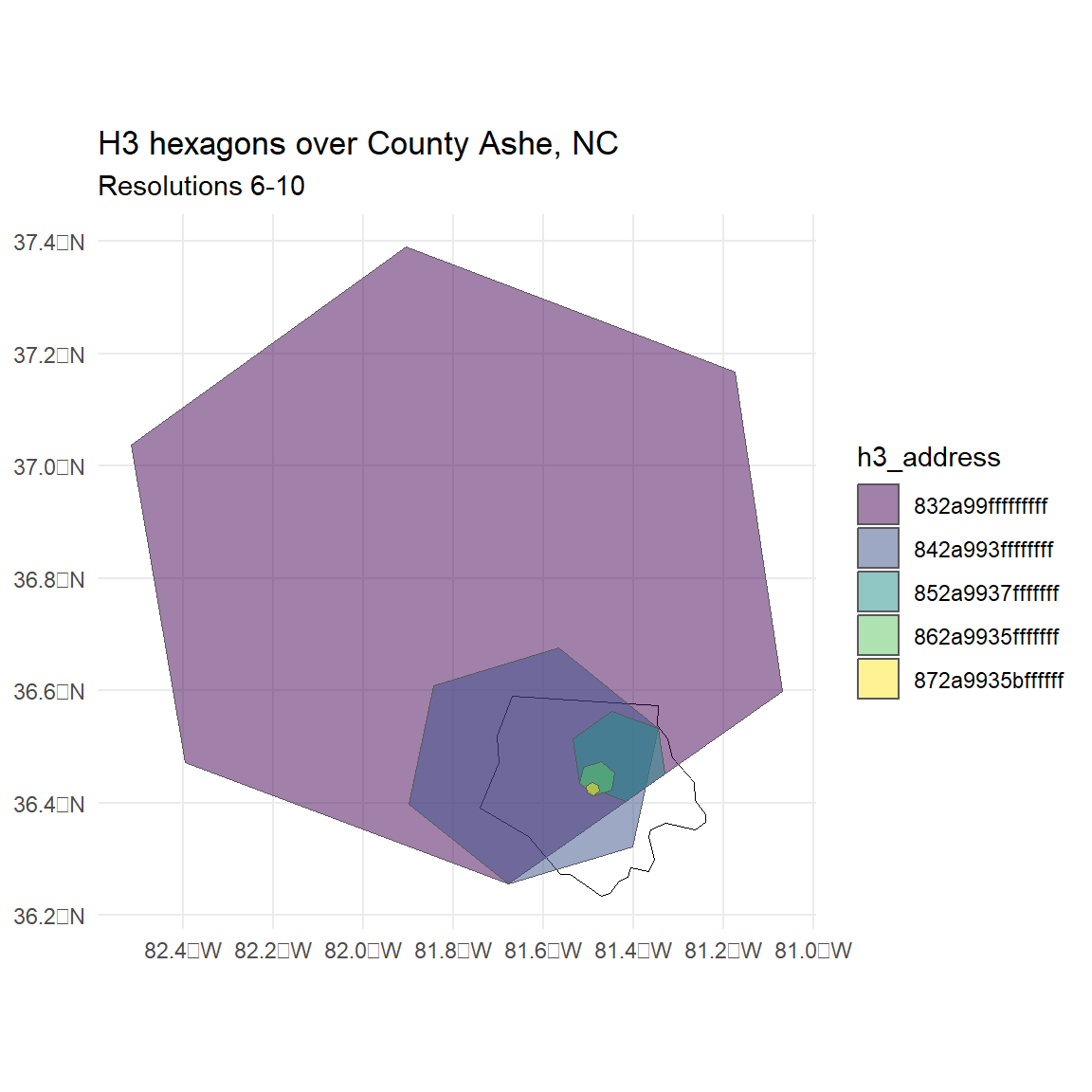Hopefully the above plot gives a sense of the large scale changes between resolution levels - each level is seven times more detailed than the last.

H3 address validity checks are done with `is_valid()`:

``````is_valid(h3_address = '8abe8d12acaffff')
#>  TRUE
#>  FALSE``````

You can check whether an address refers to one of the pentagons that occur on icosahedron corners at each resolution with `is_pentagon()`. This is relevant where subsequent area or distance calculations will be carried out. All of the pentagon indices for a given resolution can be identified using `get_pentagons()`.

``````# is the following address a pentagon?
#>  FALSE

get_pentagons(res = 8)
#> []
#>   "8808000001fffff" "881c000001fffff" "8830000001fffff" "884c000001fffff"
#>   "8862000001fffff" "8874000001fffff" "887e000001fffff" "8890000001fffff"
#>   "88a6000001fffff" "88c2000001fffff" "88d6000001fffff" "88ea000001fffff"

ggplot() +
geom_sf(data = cell_to_polygon(get_pentagons(8)[]), fill = NA) +
theme_void()``````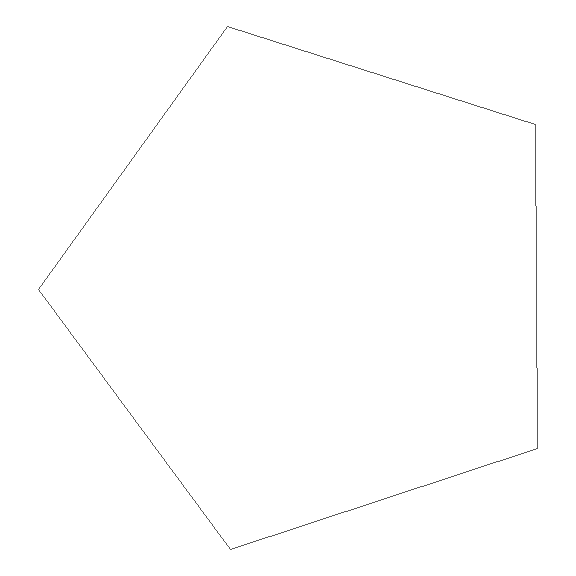`is_rc3()` checks whether an H3 address has a resolution with Class III orientation. This refers to the orientation of the hex grid relative to the next coarser resolution.

``````is_rc3(h3_address = '8abe8d12acaffff')
#>  FALSE``````

The number of the base (resolution-0) cell for any H3 address can be retrieved with `get_base_cell()`. These run from 0 at the North Pole to 121 at the South.

``````get_base_cell(h3_address = '8abe8d12acaffff')
#>  95``````

The triangular icosahedron face (or faces) that a cell belongs to can also be retrieved with `get_faces()`. These run 1-20, North to South.

``````get_faces(h3_address = '8abe8d12acaffff')
#>  15``````

Lastly, the resolution of an H3 address can be retrieved with `get_res()`.

``````get_res(h3_address = '8abe8d12acaffff')
#>  10``````

## Neighbour Algorithms

As the H3 grid system is hierarchical, addresses have parents and children. A parent address is the one that contains the given address at a coarser resolution. A child address is contained by the given address. Parents and children can be requested at any resolution above and below the input, respectively.

``````# input is res 10:
get_parent(h3_address = '8abe8d12acaffff', res = 6)
#>  "86be8d12fffffff"
# input is res 6:
get_children(h3_address = '86be8d12fffffff', res = 7)
#> []
#>  "87be8d128ffffff" "87be8d129ffffff" "87be8d12affffff" "87be8d12bffffff"
#>  "87be8d12cffffff" "87be8d12dffffff" "87be8d12effffff"

ggplot() +
geom_sf(data = cell_to_polygon('86be8d12fffffff'), fill = NA) +
res = 7)[]),
fill = 'red', alpha = 0.5 ) +
theme_void()``````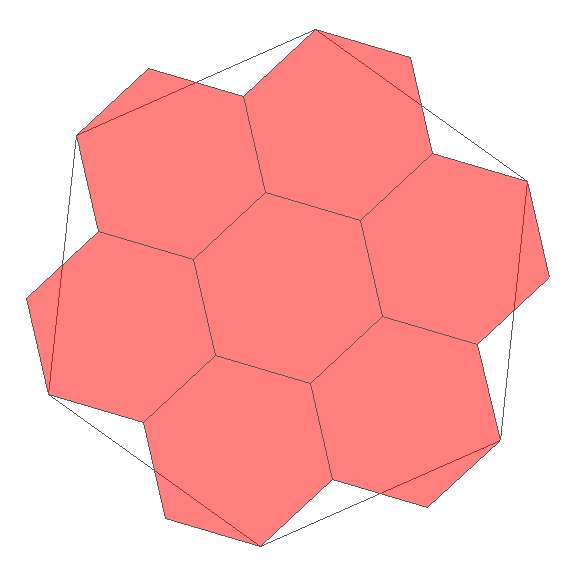The number of addresses returned for each request is `7 ^ (parent_res - child_res)`, so jumping three levels will return 343 addresses for a single input, and that’s about 41 kb.

To return only the central child for a given address, use `get_centerchild()`:

``````# input is res 6:
get_centerchild(h3_address = '86be8d12fffffff', res = 7)
#>  "87be8d128ffffff"

ggplot() +
geom_sf(data = cell_to_polygon('86be8d12fffffff'), fill = NA) +
geom_sf(data = cell_to_polygon(get_centerchild('86be8d12fffffff', 7)),
fill = 'red') +
geom_sf(data = cell_to_polygon(get_centerchild('86be8d12fffffff', 8)),
fill = 'blue') +
theme_void()``````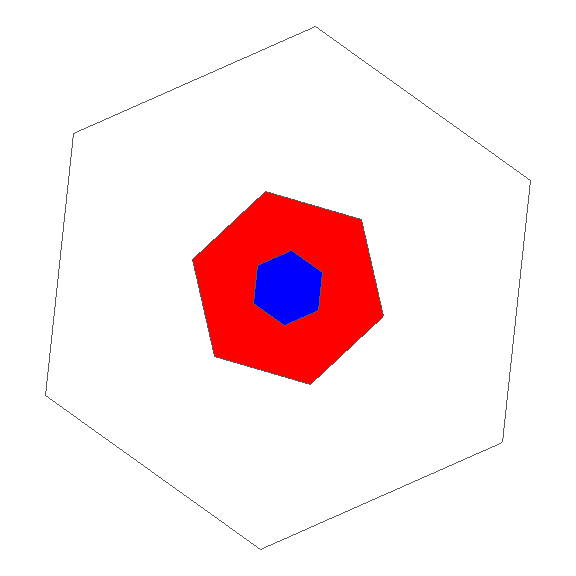Within the same resolution, addresses within n ‘steps’ from a central address (a ‘patch’ of hexagons) can be retrieved with `get_disk()` or `get_disk_list()`. The latter function returns an output where addresses are listed separately for each step away from the input. The input address is included in the output.

``````get_disk(h3_address = '86be8d12fffffff', ring_size = 2)
#> []
#>   "86be8d12fffffff" "86be8d127ffffff" "86be8d107ffffff" "86be8d10fffffff"
#>   "86be8d177ffffff" "86be8d8dfffffff" "86be8d8d7ffffff" "86be8d88fffffff"
#>   "86be8d89fffffff" "86be8d137ffffff" "86be8d117ffffff" "86be8d11fffffff"
#>  "86be8d027ffffff" "86be8d157ffffff" "86be8d147ffffff" "86be8d167ffffff"
#>  "86be8d8cfffffff" "86be8d8c7ffffff" "86be8d8f7ffffff"

get_disk_list(h3_address = '86be8d12fffffff', ring_size = 2)
#> []
#> [][]
#>  "86be8d12fffffff"
#>
#> [][]
#>  "86be8d127ffffff" "86be8d107ffffff" "86be8d10fffffff" "86be8d177ffffff"
#>  "86be8d8dfffffff" "86be8d8d7ffffff"
#>
#> [][]
#>   "86be8d88fffffff" "86be8d89fffffff" "86be8d137ffffff" "86be8d117ffffff"
#>   "86be8d11fffffff" "86be8d027ffffff" "86be8d157ffffff" "86be8d147ffffff"
#>   "86be8d167ffffff" "86be8d8cfffffff" "86be8d8c7ffffff" "86be8d8f7ffffff"``````

A ring of addresses at exactly n steps is obtained with `get_ring()`.

``````get_ring(h3_address = '86be8d12fffffff', ring_size = 2)
#> []
#>   "86be8d8f7ffffff" "86be8d88fffffff" "86be8d89fffffff" "86be8d137ffffff"
#>   "86be8d117ffffff" "86be8d11fffffff" "86be8d027ffffff" "86be8d157ffffff"
#>   "86be8d147ffffff" "86be8d167ffffff" "86be8d8cfffffff" "86be8d8c7ffffff"``````

These address lists can all be spatialised with `cell_to_multipolygon()`, which returns the polygonised outline of a collection of H3 addresses.

``````disk <- get_disk(h3_address = '86be8d12fffffff', ring_size = 2)

ring <- get_ring(h3_address = '86be8d12fffffff', ring_size = 5)

patch_sf <- cells_to_multipolygon(disk, simple = FALSE)
donut_sf <- cells_to_multipolygon(ring, simple = FALSE)

ggplot() +
geom_sf(data = patch_sf, alpha = 0.5) +
theme_minimal() +
geom_sf(data = donut_sf, alpha = 0.5, fill = 'red') +
theme_void()``````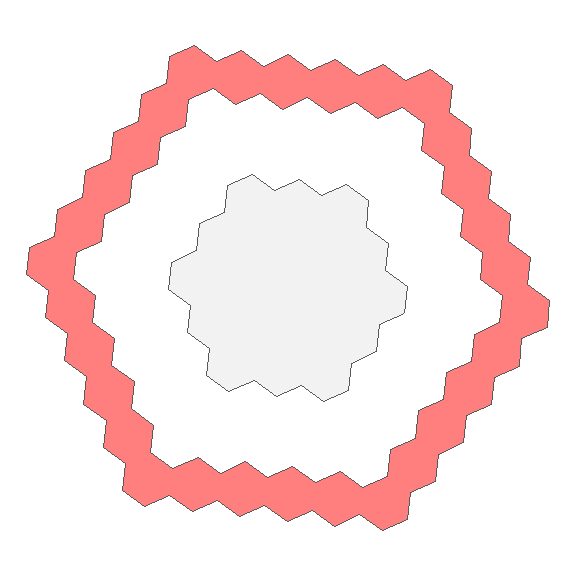But it may be more interesting to use `cell_to_polygon()`

``````disk_singles <- cell_to_polygon(unlist(disk, use.names = FALSE), simple = FALSE)
ring_singles <- cell_to_polygon(unlist(ring, use.names = FALSE), simple = FALSE)

ggplot(disk_singles) +
geom_sf(aes(fill = 1:nrow(disk_singles)), show.legend = FALSE) +
scale_fill_viridis_c() +
theme_minimal() +
theme_void()``````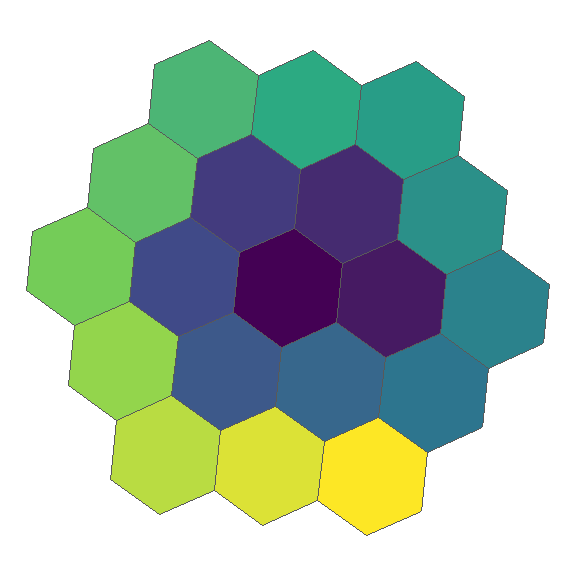``````
ggplot(ring_singles) +
geom_sf(aes(fill = 1:nrow(ring_singles)), show.legend = FALSE) +
scale_fill_viridis_c() +
theme_minimal() +
theme_void()``````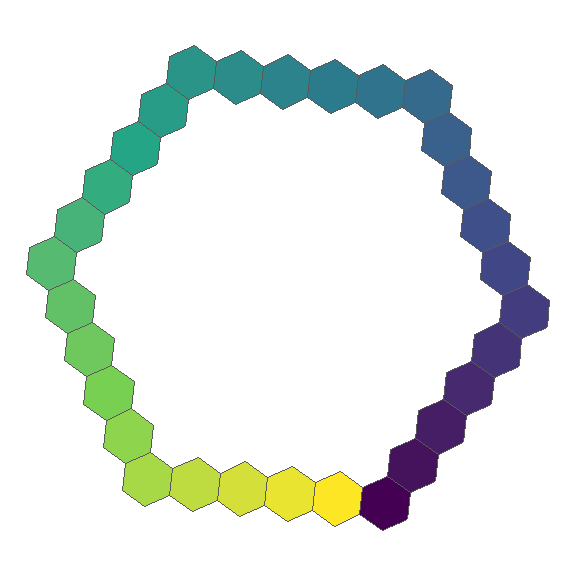`polygon_to_cells()` will return all the h3 addresses whose centers intersect a given polygon. Multipolygons are supported as well.

``````ashe <- st_transform(nc[1, ], crs = 4326)
ashe_7 <- polygon_to_cells(ashe, res = 7, simple = FALSE)
ashe_7 <- cell_to_polygon(unlist(ashe_7\$h3_addresses), simple = FALSE)

ggplot() +
geom_sf(data = ashe, fill = NA) +
geom_sf(data = ashe_7, fill = NA, colour = 'red') +
ggtitle('Resolution 7 hexagons', subtitle = 'County Ashe, NC') +
theme_minimal() +
coord_sf()``````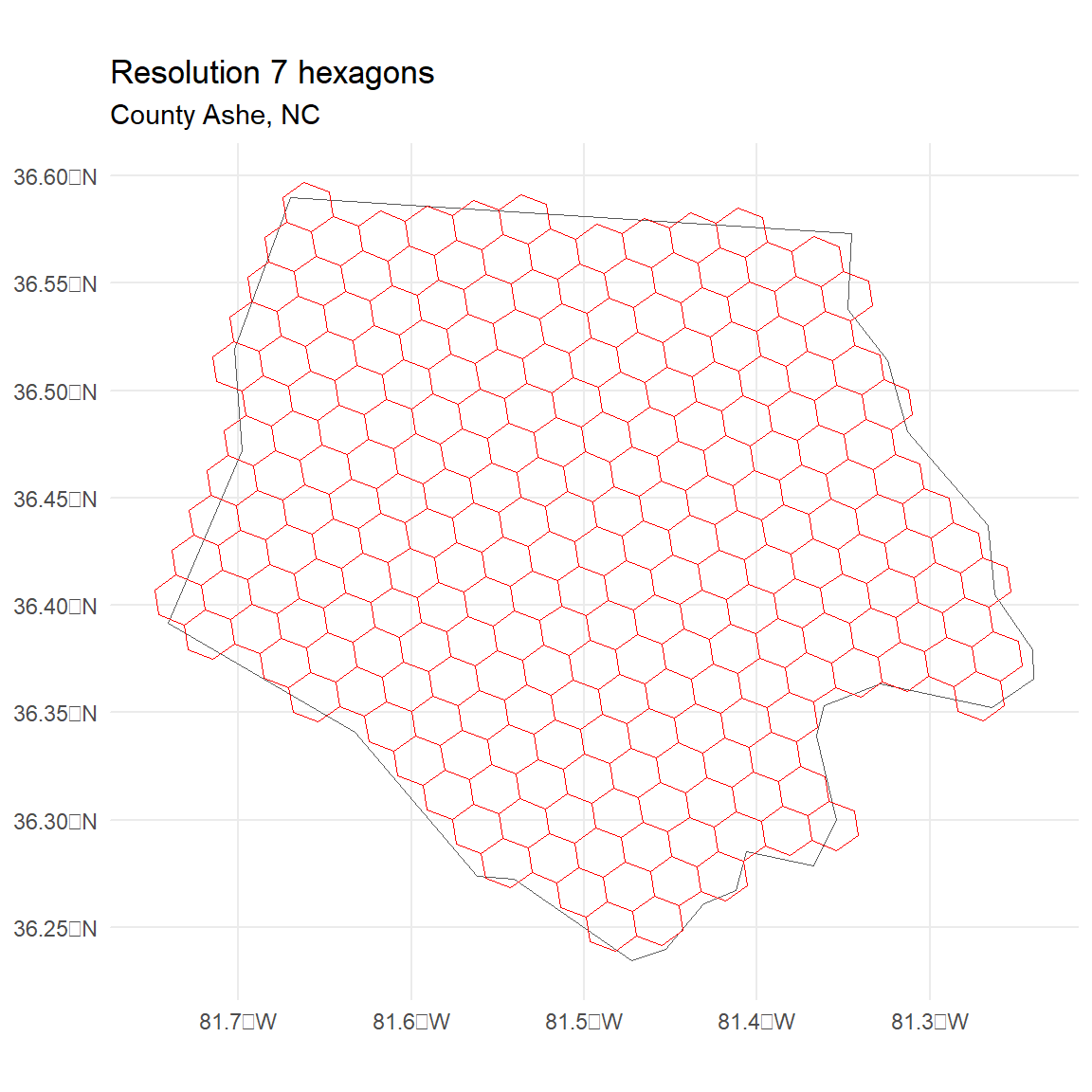A representation like this can be ‘compacted’ with `compact()`.

``````ashe_comp <- compact(ashe_7\$h3_address)
ashe_comp <- cell_to_polygon(ashe_comp, simple = FALSE)

ggplot() +
geom_sf(data = ashe, fill = NA) +
geom_sf(data = ashe_comp, fill = NA, colour = 'red') +
ggtitle('Compacted hexes from resolution 7', subtitle = 'County Ashe, NC') +
theme_minimal() +
coord_sf()``````